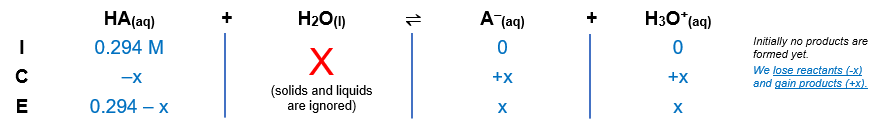# Problem: Determine the Ka of an acid whose 0.294 M solution has a pH of 2.80.A) 4.9 x 10-7 B ) 1.2x 10-5 C) 8.5 x 10-6 D) 5.4 10-3 E) 27

###### FREE Expert Solution

Let HA → weak acid

Equilibrium reaction:        HA(aq) + H2O(l)  A-(aq) + H3O+(aq)$\overline{){{\mathbf{K}}}_{{\mathbf{a}}}{\mathbf{=}}\frac{\mathbf{products}}{\mathbf{reactants}}}\phantom{\rule{0ex}{0ex}}{\mathbf{K}}_{\mathbf{a}}\mathbf{=}\frac{\left[{\mathbf{A}}^{\mathbf{-}}\right]\left[{\mathbf{H}}_{\mathbf{3}}{\mathbf{O}}^{\mathbf{+}}\right]}{\left[\mathbf{HA}\right]}$

Solids and liquids are not included in the expression

${\mathbf{K}}_{\mathbf{a}}\mathbf{=}\frac{\left[\mathbf{x}\right]\left[\mathbf{x}\right]}{\left[\mathbf{0}\mathbf{.}\mathbf{294}\mathbf{-}\mathbf{x}\right]}\phantom{\rule{0ex}{0ex}}\overline{){{\mathbf{K}}}_{{\mathbf{a}}}{\mathbf{=}}\frac{{\mathbf{x}}^{\mathbf{2}}}{\mathbf{0}\mathbf{.}\mathbf{294}\mathbf{-}\mathbf{x}}}$

94% (310 ratings)###### Problem Details

Determine the Ka of an acid whose 0.294 M solution has a pH of 2.80.

A) 4.9 x 10-7

B ) 1.2x 10-5

C) 8.5 x 10-6

D) 5.4 10-3

E) 27